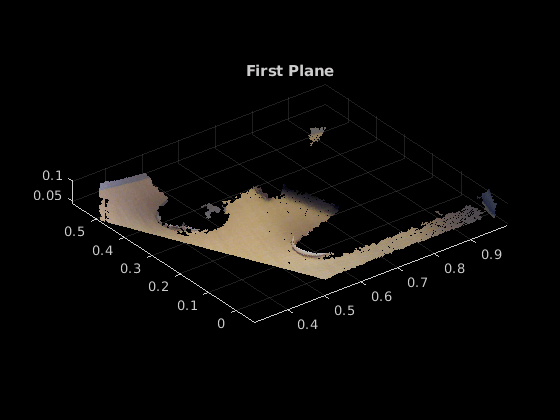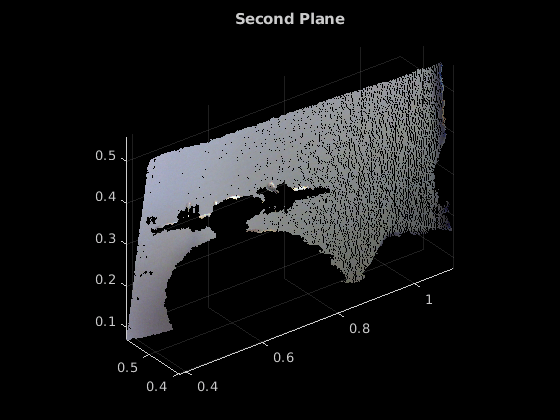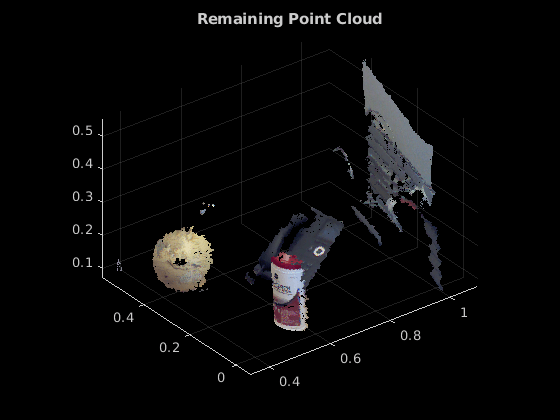planeModel

Object for storing a parametric plane model

Description

Construct and store a parametric plane model based on parameters that describe a plane.

Creation

Description

example

model = planeModel(Parameters) constructs a parametric plane model from the 1-by-4 Parameters input vector that describes a plane.

Input Arguments

expand all

Plane parameters, specified as a 1-by-4 vector. This input specifies the Parameters property. The four parameters [a,b,c,d] describe the equation for a plane:

$ax+by+cz+d=0$

Properties

expand all

Plane model parameters, stored as a 1-by-4 vector. These parameters are specified by the Parameters input argument.

Normal vector of the plane, stored as a 1-by-3 vector. The [a,b,c] vector specifies the unnormalized normal vector of the plane.

Object Functions

 plot Plot plane in a figure window normalRotation Compute transform for rotation of a normal to a plane

Examples

collapse all

Display and label the point cloud.

figure
pcshow(ptCloud)
xlabel('X(m)')
ylabel('Y(m)')
zlabel('Z(m)')
title('Original Point Cloud')Set the maximum point-to-plane distance (2cm) for plane fitting.

maxDistance = 0.02;

Set the normal vector of the plane.

referenceVector = [0,0,1];

Set the maximum angular distance to 5 degrees.

maxAngularDistance = 5;

Detect the first plane, the table, and extract it from the point cloud.

[model1,inlierIndices,outlierIndices] = pcfitplane(ptCloud,...
maxDistance,referenceVector,maxAngularDistance);
plane1 = select(ptCloud,inlierIndices);
remainPtCloud = select(ptCloud,outlierIndices);

Set the region of interest to constrain the search for the second plane, left wall.

roi = [-inf,inf;0.4,inf;-inf,inf];
sampleIndices = findPointsInROI(remainPtCloud,roi);

Detect the left wall and extract it from the remaining point cloud.

[model2,inlierIndices,outlierIndices] = pcfitplane(remainPtCloud,...
maxDistance,'SampleIndices',sampleIndices);
plane2 = select(remainPtCloud,inlierIndices);
remainPtCloud = select(remainPtCloud,outlierIndices);

Plot the two planes and the remaining points.

figure
pcshow(plane1)
title('First Plane')figure
pcshow(plane2)
title('Second Plane')figure
pcshow(remainPtCloud)
title('Remaining Point Cloud')